# Chern character

(diff) ← Older revision | Latest revision (diff) | Newer revision → (diff)

A characteristic class defining a ring homomorphism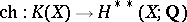. For a one-dimensional bundlethere is the identity, where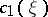is the rational Chern class. This identity, together with the requirement that the class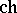define a homomorphism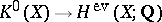, uniquely determines the class. There is a commutative diagram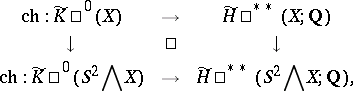in which the vertical arrows denote the periodicity operator and the dual suspension. Let the mappingcoincide with the composition(here "+" denotes the functor from the category of topological spaces into the category of pointed spaces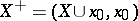. One obtains a functorial transformation, and this induces a transformation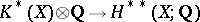, which is a natural isomorphism of-graded rings.

If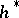is a generalized cohomology theory in which the Chern classesare defined, then for one-dimensional bundlesthe generalized Chern characteris defined by the formula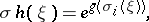whereis the logarithm of the formal group corresponding to the theory. By the splitting lemma one can define a natural ring homomorphism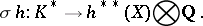For a generalized cohomology theorythere exists a unique natural isomorphism of graded groups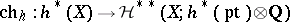, which forcoincides with the mappingHereThe mapping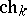, where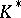is a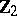-graded-theory, coincides with the Chern character. The natural transformation functor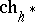is called the Chern–Dold character.

Letbe the unitary cobordism theoryand letbe the space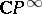. The ring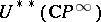is isomorphic to the ring of formal power series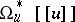, whereand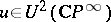is the orientation of the bundle. Analogously, the ring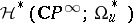is isomorphic to, where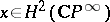is the orientation of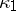. The formal power series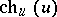is the functional inverse of the Mishchenko series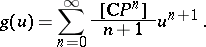For references see Chern class.